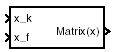# Interpolate Matrix(x)

Return interpolated matrix for given input

• Library:
• Aerospace Blockset / GNC / Control

•## Description

The Interpolate Matrix(x) block interpolates a one-dimensional array of matrices. The block assumes a one-dimensional array as defined in Algorithms.

The matrix to be interpolated must be three dimensional, the first two dimensions corresponding to the matrix at each value of x. For example, if you have three matrices A, B, and C defined at `x = 0`, `x = 0.5`, and `x = 1.0`, then the input matrix is given by

`matrix(:,:,1) = A;`

`matrix(:,:,2) = B;`

`matrix(:,:,3) = C;`

## Limitations

This block must be driven from the Prelookup block.

## Ports

### Input

expand all

Interpolation index i, specified as a scalar.

Data Types: `single` | `double` | `int8` | `int16` | `int32` | `uint8` | `uint16` | `uint32` | `Boolean` | `fixed point` | `enumerated` | `bus`

Interpolation fraction λ, specified as a scalar.

Data Types: `single` | `double` | `int8` | `int16` | `int32` | `uint8` | `uint16` | `uint32` | `Boolean` | `fixed point` | `enumerated` | `bus`

### Output

expand all

Interpolated matrix, specified as a matrix.

Data Types: `single` | `double` | `int8` | `int16` | `int32` | `uint8` | `uint16` | `uint32` | `Boolean` | `fixed point` | `enumerated` | `bus`

## Parameters

expand all

Matrix to be interpolated, with three indices and the third index labeling the interpolating values of x.

#### Programmatic Use

 Block Parameter: `matrix` Type: character vector Values: matrix Default: `'matrix'`

## Algorithms

This one-dimensional case assumes a matrix M is defined at a discrete number of values of an independent variable

x = [ x1x2x3 ... xixi+1 ... xn ].

Then for xi < x < xi+1, the block output is given by

`$\left(1-\lambda \right)M\left({x}_{i}\right)+\lambda M\left({x}_{i+1}\right)$`

where the interpolation fraction is defined as

`$\lambda =\left(x-{x}_{i}\right)/\left({x}_{i+1}-{x}_{i}\right)$`

## Version History

Introduced before R2006a